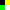# Reading PNG files (SOLVED)

#1

Hello,
I am trying to create some kind of photoshop and first I need to know info about each pixel of an image, if possible in format rgba. I tried to work with the PNG-extension, but I only found a way to find out the height and width of the image in pixels…

0 Likes

#2

You should be able to use png.decode_rgba() to get the image as a Defold buffer. You can then read pixels from the buffer.

The Draw Pixels extension and drawpixels.color(buffer, x, y) should give you an idea of how this can be achieved:

Also check the buffer API to learn how to manipulate buffers from Lua:

4 Likes

#4

Thank you, but I don’t have much experience with Defold and I just don’t know how to use the command in a code… I wrote this code, but it does not work properly…

``````function init(self)
width = 256
height = 256
local size = 128
self.image = buffer.create( size * size, { {name=hash(“rgba”),
type=buffer.VALUE_TYPE_UINT8, count=4 } })
self.imagestream = buffer.get_stream(self.image, hash(“rgba”))

local f = io.open("Images\\kraken.png", "rb")
local bytes = f:read("*a")

local buf, w, h = png.decode_rgba(bytes, true)
print(buf)

end
``````

And this is the output:
`DEBUG:SCRIPT: buffer.buffer(count = 2529120, { hash("pixels"), buffer.VALUE_TYPE_UINT8, 4 })`

0 Likes

#5

Your “self.image” and “buf” are two different buffers.
“buf” is the raw data buffer containing the argb(a) values.

As seen from the printout, the stream name is “pixels”, so to access the bytes:

local bytes = buffer.get_stream(buf, hash(“pixels”))

1 Like

#6

So I don’t have to use the “self.image” buffer?
I’m afraid I still don’t understand it well. I tried to change my code, but the printout looks similar…

``````function init(self)
local f = io.open("Images\\kraken.png", "rb")
local bytes = f:read("*a")

local buf, w, h = png.decode_rgba(bytes, true)
local bytes = buffer.get_stream(buf, hash("pixels"))
print(bytes)
end
``````

Output: `DEBUG:SCRIPT: buffer.bufferstream({ hash("pixels"), buffer.VALUE_TYPE_UINT8, 4 })`

0 Likes

#7

Yes, the “print(bytes)” will only printout some meta data about the buffer.
There’s no point in printing out all the bytes from the image, right?

It’s probably more useful to loop over and access the pixels that you want?
See the example from the documentation for how to do that with an stream named “rgb”.

1 Like

#8

Do you think you could send me a code sample?

0 Likes

#9
``````local function get_pixel_rgba(pixels, width, x, y)
local index = y * width * 4 + x * 4 + 1
local r = pixels[index + 0]
local g = pixels[index + 1]
local b = pixels[index + 2]
local a = pixels[index + 3]
return r,g,b,a
end

function init(self)
local f = io.open("test.png", "rb")
local image = f:read("*a")

local buf, w, h = png.decode_rgba(image, true)
local pixels = buffer.get_stream(buf, hash("pixels"))

print(get_pixel_rgba(pixels, w, 2, 8))
end
``````

With this test imageyou’ll the following output:

``````DEBUG:SCRIPT: 0	0	0	255
``````

At pixel position [2,8] there is a black pixel (rgba: 0,0,0,255).

4 Likes

#10

Finally works! Thank you @britzl, @Mathias_Westerdahl !!!

This is the final code for all pixels:

``````local function get_pixel_rgba(pixels, width, x, y)
local index = y * width * 4 + x * 4 + 1
local r = pixels[index + 0]
local g = pixels[index + 1]
local b = pixels[index + 2]
local a = pixels[index + 3]
return r,g,b,a
end

function init(self)
local file = io.open("images\\kraken.png", "rb")
local file = file:read("*a")

local buf, w, h = png.decode_rgba(file, true)
local pixels = buffer.get_stream(buf, hash("pixels"))
for x = 0, w - 1 do
for y = 0, h - 1 do
print(get_pixel_rgba(pixels, w, x, y))
end
end
end``````
6 Likes